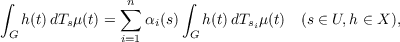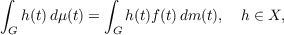#### Vol. 15, No. 4, 1965

 Download this articleFor screen For printingRecent Issues Vol. 323: 1  2 Vol. 322: 1  2 Vol. 321: 1  2 Vol. 320: 1  2 Vol. 319: 1  2 Vol. 318: 1  2 Vol. 317: 1  2 Vol. 316: 1  2Online Archive Volume: Issue:The Journal Subscriptions Editorial Board Officers Contacts Submission Guidelines Submission Form Policies for Authors ISSN: 1945-5844 (e-only) ISSN: 0030-8730 (print) Special Issues Author Index To Appear Other MSP Journals
Almost invariant measures

### Ronald John Larsen

Vol. 15 (1965), No. 4, 1295–1305
##### Abstract

Let μ be a regular complex-valued Borel measure on a locally compact topological (LC) group G which is finite on compact sets; and for each s G define the measure Tsμ by Tsμ(E) = μ(E + s), E Bc(G) the collection of all Borel subsets of G with compact closure. If f is a function on G then for each s G we set Tsf(t) = f(t + s), t G. Let X be a translation invariant subspace of C0(G), the space of continuous complex-valued functions on G which vanish at infinity, i.e., a subspace such that f X implies Tsf X, s G; and let U be an open symmetric neighborhood of zero in G. Then we shall say μ acts U-almost invariantly on X if G|h(t)|d|μ|(t) < , h X, andwhere s1,s2,,sn are fixed elements of U. We shall say μ is a U-almost invariant measure on G if {Tsμs U} spans a finite dimensional space of measures. When U = G we shall say μ acts almost invariantly and μ is an almost invariant measure, respectively. The main results of this paper show that if μ acts U-almost invariantly on X then there exists some continuous function f such thatwhere dm is right invariant Haar measure on G; and that μ is a U-almost invariant measure if and only if there exists a continuous f such that (t) = f(t)dm(t) and {Tsfs U} spans a finite dimensional space of functions.

Primary: 28.75
##### Milestones